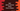# How to add and remove items from a Queue in Dart## How to add and remove items from a Queue in Dart:

The Queue class of Dart can be used to create a queue. There are different methods available to add and remove items from a queue. In this post, we will learn how to create a Queue and how to remove and add items from it with different examples.

### How to create a queue with elements:

We can create an empty queue with the Queue() constructor. There is one more constructor available to create a queue with all elements from an iterable. It is:

``Queue.from(Iterable it)``

You can also use Queue.of:

``Queue.of(Iterable<E> it)``
``````import 'dart:collection';

void main() {
var q = Queue.of([1, 2, 3, 4]);
print(q);
}``````

It keeps adding the elements of the iterable to the end of the queue. It will print:

``{1, 2, 3, 4}``

The add() method takes one single element and adds it to the queue. It is defined as:

``add(E e) -> void``

Let’s try it with an example:

``````import 'dart:collection';

void main() {
var q = Queue();
print(q);
}``````

It will add 1 and 2 to the queue. It will print:

``{1, 2}``

The addAll method adds all elements of an iterable to the end of the queue. It is defined as:

``addAll(Iterable<E> it) -> void``

Example:

``````import 'dart:collection';

void main() {
var q = Queue();

print(q);
}``````

Output:

``{1, 2, 3, 4, 5, 6}``

### Add elements to the beginning and end of a queue:

The Queue class provides two methods to add an element to the start and end of a queue. These are:

``````addFirst(E e) -> void

For example,

``````import 'dart:collection';

void main() {
var q = Queue.of([1, 2, 3, 4]);

print(q);
}``````

It created the queue q with Queue.of and added 0 to the start and 5 to the end.

Output:

``{0, 1, 2, 3, 4, 5}``

### Remove all elements from a queue:

The clear() method removes all elements of a queue:

``````import 'dart:collection';

void main() {
var q = Queue.of([1, 2, 3, 4]);
q.clear();

print(q);
}``````

It will print:

``{}``

### Remove a single item from a queue:

We can pass an object to the remove method to remove a single instance from the queue.

``````import 'dart:collection';

void main() {
var q = Queue.of([1, 2, 3, 4]);
q.remove(2);

print(q);
}``````

It will print:

``{1, 3, 4}``

It removes a single instance of the value. If we have two instances, it will remove only one:

``````import 'dart:collection';

void main() {
var q = Queue.of([1, 2, 3, 4, 2]);
q.remove(2);

print(q);
}``````

It will remove only the first 2:

``{1, 3, 4, 2}``

### Remove the first and the last element of a queue:

We can use the following methods to remove the first and the last elements of a queue:

``````removeFirst() -> E

removeLast() -> E``````

For example:

``````import 'dart:collection';

void main() {
var q = Queue.of([1, 2, 3, 4, 5]);
q.removeFirst();
q.removeLast();

print(q);
}``````

It will remove 1 and 5.

``{2, 3, 4}``

### Remove specific elements with removeWhere:

The removeWhere function takes one function as its parameter and removes all elements for those the function returns true. Its syntax is:

``removeWhere(bool fn(E e)) -> void``

Example:

``````import 'dart:collection';

void main() {
var q = Queue.of([1, 2, 3, 4, 5, 6, 7, 8, 9, 10]);
q.removeWhere((e) => e % 2 == 0);

print(q);
}``````

This program will remove all even items from the queue.

``{1, 3, 5, 7, 9}``